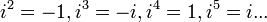# Talk:271: Powers of One

I want a "Powers of$i$" documentary. 172.68.132.95 08:21, 27 February 2019 (UTC)
Basiclly it will in each iteration rotate image 90 degrees. /*$i^2 = -1 , i^3 = -i , i^4 = 1 , i^5 = i ...$